• 1.1 PIL：Python图像处理类库 1.1.1 转换图像格式——save()函数 1.1.2 创建缩略图 1.1.3 复制并粘贴图像区域 1.1.4 调整尺寸和旋转 1.2 Matplotlib库 1.2.1 画图、描点和线 1.2.2 图像轮廓和直方图 1.2.3 交互式...


第1章 基本的图像操作和处理
1.1 PIL：Python图像处理类库
1.1.1 转换图像格式——save()函数
1.1.2 创建缩略图
1.1.3 复制并粘贴图像区域
1.1.4 调整尺寸和旋转

1.2 Matplotlib库
1.2.1 画图、描点和线
1.2.2 图像轮廓和直方图
1.2.3 交互式标注

1.3 NumPy库
1.3.1 图像数组表示
1.3.2 灰度变换
1.3.3 图像缩放
1.3.4 直方图均衡化
1.3.5 图像平均
1.3.5 对图像进行主成分分析
1.3.6 Pickle模块

1.4 SciPy
1.4.1 图像模糊
1.4.2 图像导数
1.4.3 形态学：对象计数
1.4.4 有用的SciPy模块

1.5 高级示例：图像去噪

第1章 基本的图像操作和处理

1.1 PIL：Python图像处理类库

PIL（Python Imaging Library，图像处理库）提供了通用的图像处理功能，以及大量有用的基本图像操作。PIL库已经集成在Anaconda库中，推荐使用Anaconda，简单方便，常用库都已经集成。

PIL简明教程

读入一副图像：

from PIL import Image
from pylab import *

# 添加中文字体支持
from matplotlib.font_manager import FontProperties
font = FontProperties(fname=r"c:\windows\fonts\SimSun.ttc", size=14)
figure()

pil_im = Image.open('E:\python\Python Computer Vision\Image data\empire.jpg')
gray()
subplot(121)
title(u'原图',fontproperties=font)
axis('off')
imshow(pil_im)

pil_im = Image.open('E:\python\Python Computer Vision\Image data\empire.jpg').convert('L')
subplot(122)
title(u'灰度图',fontproperties=font)
axis('off')
imshow(pil_im)

show()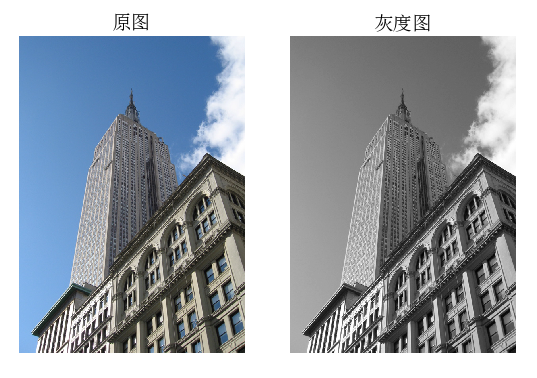1.1.1 转换图像格式——save()函数

from PCV.tools.imtools import get_imlist #导入原书的PCV模块
from PIL import Image
import os
import pickle

filelist = get_imlist('E:/python/Python Computer Vision/test jpg/') #获取convert_images_format_test文件夹下的图片文件名(包括后缀名)
imlist = open('E:/python/Python Computer Vision/test jpg/imlist.txt','wb+')
#将获取的图片文件列表保存到imlist.txt中
pickle.dump(filelist,imlist) #序列化
imlist.close()

for infile in filelist:
outfile = os.path.splitext(infile) + ".png" #分离文件名与扩展名
if infile != outfile:
try:
Image.open(infile).save(outfile)
except IOError:
print ("cannot convert", infile)


其中，test jpg文件夹是作者自己建立的文件夹，存放测试的**.jpg图像，源代码证添加了部分代码以便将获取的图像文件名保存下来，同时将所有的图像转化为.png格式，运行程序后的结果如下：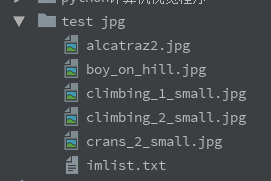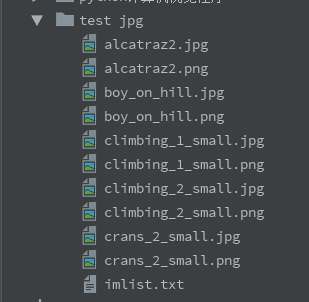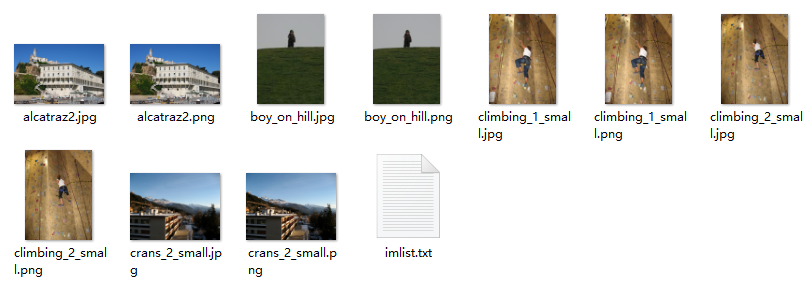PIL中的open()函数用于创建PIL图像对象，sace()方法用于保存如下到指定文件名的文件夹，上述过程将后缀变为.png，但文件名不变

1.1.2 创建缩略图

利用PIL可以很容易的创建缩略图，设置缩略图的大小，并用元组保存起来，调用thumnail()方法即可生成缩略图。创建缩略图的代码见下面。
例如创建最长边为128像素的缩略图，可以使用：

pil_im.thumbnail((128,128))

1.1.3 复制并粘贴图像区域

调用crop()方法即可从一幅图像中进行区域拷贝，拷贝出区域后，可以对区域进行旋转等变换。

box=(100,100,400,400)
region=pil_im.crop(box)

目标区域由四元组来指定，坐标依次为（左，上，右，下），PIL中指定坐标系的左上角坐标为（0，0），可以旋转后利用paste()放回去，具体实现如下：

region=region.transpose(Image.ROTATE_180)
pil_im.paste(region,box)

1.1.4 调整尺寸和旋转

调整尺寸：利用resize()方法，参数是一个元组，用来指定新图像的大小：

out=pil_im.resize((128,128))

旋转：利用rotate()方法，逆时针方式表示角度

out=pil_im.rotate(45)

上述操作的代码如下：

from PIL import Image
from pylab import *

# 添加中文字体支持
from matplotlib.font_manager import FontProperties

font = FontProperties(fname=r"c:\windows\fonts\SimSun.ttc", size=14)
figure()

# 显示原图
pil_im = Image.open('E:/python/Python Computer Vision/Image data/empire.jpg')
print(pil_im.mode, pil_im.size, pil_im.format)
subplot(231)
title(u'原图', fontproperties=font)
axis('off')
imshow(pil_im)

# 显示灰度图
pil_im = Image.open('E:/python/Python Computer Vision/Image data/empire.jpg').convert('L')
gray()
subplot(232)
title(u'灰度图', fontproperties=font)
axis('off')
imshow(pil_im)

# 复制并粘贴区域
pil_im = Image.open('E:/python/Python Computer Vision/Image data/empire.jpg')
box = (100, 100, 400, 400)
region = pil_im.crop(box)
region = region.transpose(Image.ROTATE_180)
pil_im.paste(region, box)
subplot(233)
title(u'复制粘贴区域', fontproperties=font)
axis('off')
imshow(pil_im)

# 缩略图
pil_im = Image.open('E:/python/Python Computer Vision/Image data/empire.jpg')
size = 128, 128
pil_im.thumbnail(size)
print(pil_im.size)
subplot(234)
title(u'缩略图', fontproperties=font)
axis('off')
imshow(pil_im)
pil_im.save('E:/python/Python Computer Vision/Image data/empire thumbnail.jpg')# 保存缩略图

#调整图像尺寸
pil_im=Image.open('E:/python/Python Computer Vision/Image data/empire thumbnail.jpg')
pil_im=pil_im.resize(size)
print(pil_im.size)
subplot(235)
title(u'调整尺寸后的图像',fontproperties=font)
axis('off')
imshow(pil_im)

#旋转图像45°
pil_im=Image.open('E:/python/Python Computer Vision/Image data/empire thumbnail.jpg')
pil_im=pil_im.rotate(45)
subplot(236)
title(u'旋转45°后的图像',fontproperties=font)
axis('off')
imshow(pil_im)

show()

运行结果如下：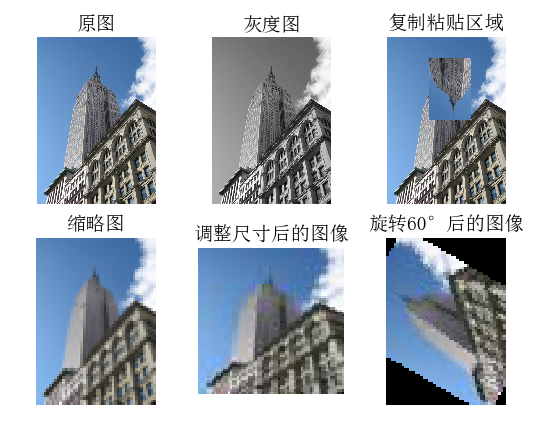1.2 Matplotlib库

当在处理数学及绘图或在图像上描点、画直线、曲线时，Matplotlib是一个很好的绘图库，它比PIL库提供了更有力的特性。

matplotlib教程

1.2.1 画图、描点和线

from PIL import Image
from pylab import *

# 添加中文字体支持
from matplotlib.font_manager import FontProperties

font = FontProperties(fname=r"c:\windows\fonts\SimSun.ttc", size=14)

# 读取图像到数组中
im = array(Image.open('E:/python/Python Computer Vision/Image data/empire.jpg'))
figure()

# 绘制有坐标轴的
subplot(121)
imshow(im)
x = [100, 100, 400, 400]
y = [200, 500, 200, 500]

# 使用红色星状标记绘制点
plot(x, y, 'r*')

# 绘制连接两个点的线（默认为蓝色）
plot(x[:2], y[:2])
title(u'绘制empire.jpg', fontproperties=font)

# 不显示坐标轴的
subplot(122)
imshow(im)
x = [100, 100, 400, 400]
y = [200, 500, 200, 500]

plot(x, y, 'r*')
plot(x[:2], y[:2])
axis('off')
title(u'绘制empire.jpg', fontproperties=font)

show()
# show()命令首先打开图形用户界面（GUI），然后新建一个窗口，该图形用户界面会循环阻断脚本，然后暂停，
# 直到最后一个图像窗口关闭。每个脚本里，只能调用一次show()命令，通常相似脚本的结尾调用。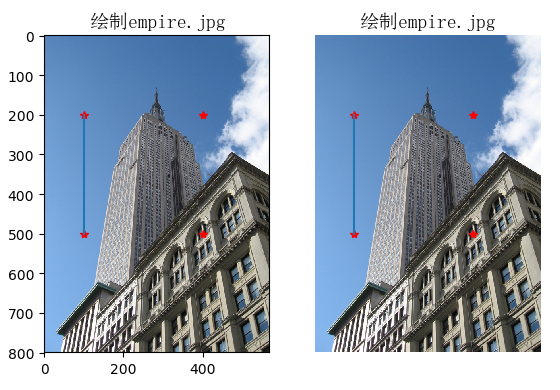绘图时还有很多可选的颜色和样式，如表1-1，1-2，1-3所示，应用例程如下：

plot(x,y)          #默认为蓝色实线
plot(x,y,'go-')    #带有圆圈标记的绿线
plot(x,y,'ks:')    #带有正方形标记的黑色虚线

表1-1 用PyLab库绘图的基本颜色格式命令

符号
颜色

‘b’
蓝色

‘g’
绿色

‘r’
红色

‘c’
青色

‘m’
品红

‘y’
黄色

‘k’
黑色

‘w’
白色

表1-2 用PyLab库绘图的基本线型格式命令

符号
线型

‘-‘
实线

‘–’
虚线

‘:’
点线

表1-3 用PyLab库绘图的基本绘制标记格式命令

符号
标记

‘.’
点

‘o’
圆圈

’s’
正方形

‘*’
星型

‘+’
加号

‘*’
叉号

1.2.2 图像轮廓和直方图

from PIL import Image
from pylab import *

# 添加中文字体支持
from matplotlib.font_manager import FontProperties

font = FontProperties(fname=r"c:\windows\fonts\SimSun.ttc", size=14)
# 打开图像，并转成灰度图像
im = array(Image.open('E:/python/Python Computer Vision/Image data/empire.jpg').convert('L'))

# 新建一个图像
figure()
subplot(121)
# 不使用颜色信息
gray()
# 在原点的左上角显示轮廓图像
contour(im, origin='image')
axis('equal')
axis('off')
title(u'图像轮廓图', fontproperties=font)

subplot(122)
# 利用hist来绘制直方图
# 第一个参数为一个一维数组
# 因为hist只接受一维数组作为输入，所以要用flatten()方法将任意数组按照行优先准则转化成一个一维数组
# 第二个参数指定bin的个数
hist(im.flatten(), 128)
title(u'图像直方图', fontproperties=font)
# plt.xlim([0,250])
# plt.ylim([0,12000])

show()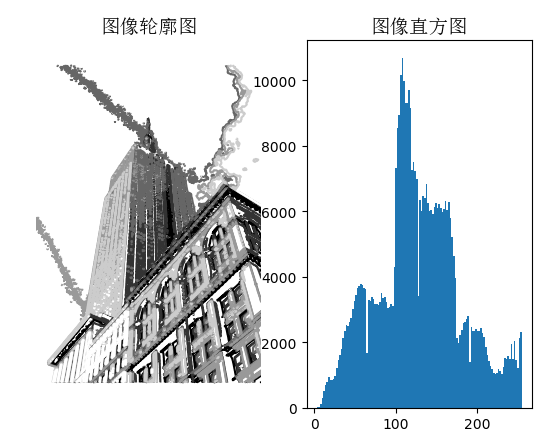1.2.3 交互式标注

有时候用户需要和应用进行交互，比如在图像中用点做标识，或者在一些训练数据中进行注释，PyLab提供了一个很简介好用的函数gitput()来实现交互式标注。

from PIL import Image
from pylab import *

im = array(Image.open('E:/python/Python Computer Vision/Image data/empire.jpg'))
imshow(im)

x = ginput(3)
print('you clicked:', x)
show()


输出：

you clicked:
[(118.4632306896458, 177.58271393177051),
(118.4632306896458, 177.58271393177051),
(118.4632306896458, 177.58271393177051)]


上面代码先读取empire.jpg图像，显示读取的图像，然后用ginput()交互注释，这里设置的交互注释数据点设置为3个，用户在注释后，会将注释点的坐标打印出来。

1.3 NumPy库

NumPy在线文档
NumPy是Python一个流行的用于科学计算包。它包含了很多诸如矢量、矩阵、图像等其他非常有用的对象和线性代数函数。

1.3.1 图像数组表示

在前面图像的示例中，我们将图像用array()函数转为NumPy数组对象，但是并没有提到它表示的含义。数组就像列表一样，只不过它规定了数组中的所有元素必须是相同的类型，除非指定以外，否则数据类型灰按照数据类型自动确定。
举例如下：

from PIL import Image
from pylab import *

im = array(Image.open('E:/python/Python Computer Vision/Image data/empire.jpg'))
print (im.shape, im.dtype)
im = array(Image.open('E:/python/Python Computer Vision/Image data/empire.jpg').convert('L'),'f')
print (im.shape, im.dtype)

输出：

(800, 569, 3) uint8
(800, 569) float32

解释：

第一个元组表示图像数组大小（行、列、颜色通道）
第二个字符串表示数组元素的数据类型，因为图像通常被编码为8位无符号整型；
1. uint8:默认类型
2. float32:对图像进行灰度化，并添加了'f'参数，所以变为浮点型

数组元素如何访问——使用下标访问

value=im[i,j,k]

多个数组元素如何发给我——使用数组切片方式访问，返回的是以指定间隔下标访问该数组的元素值

im[i,:] = im[j,:]     #将第j行的数值赋值给第i行
im[:,j] = 100         #将第i列所有数值设为100
im[:100,:50].sum()    #计算前100行、前50列所有数值的和
im[50:100,50:100]     #50~100行，50~100列，不包含第100行和100列
im[i].mean()          #第i行所有数值的平均值
im[:,-1]              #最后一列
im[-2,:]/im[-2]       #倒数第二行

1.3.2 灰度变换

将图像读入NumPy数组对象后，我们可以对它们执行任意数学操作，一个简单的例子就是图像的灰度变换，考虑任意函数f $f$$f$，它将0~255映射到自身，也就是输出区间和输入区间相同。
举例如下：

from PIL import Image
from numpy import *
from pylab import *

im=array(Image.open('E:/python/Python Computer Vision/Image data/empire.jpg').convert('L'))
print(int(im.min()),int(im.max()))

im2=255-im               #对图像进行反向处理
print(int(im2.min()),int(im2.max())) #查看最大/最小元素

im3=(100.0/255)*im+100   #将图像像素值变换到100...200区间
print(int(im3.min()),int(im3.max()))

im4=255.0*(im/255.0)**2  #对像素值求平方后得到的图像
print(int(im4.min()),int(im4.max()))

figure()
gray()
subplot(131)
imshow(im2)
axis('off')
title(r'$f(x)=255-x$')

subplot(132)
imshow(im3)
axis('off')
title(r'$f(x)=\frac{100}{255}x+100$')

subplot(133)
imshow(im4)
axis('off')
title(r'$f(x)=255(\frac{x}{255})^2$')

show()

输出：

3 255
0 252
101 200
0 255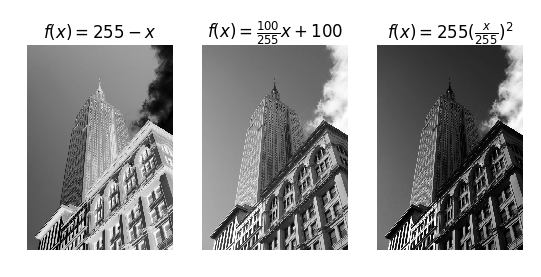array变换的相反操作可以利用PIL的fromarray()函数来完成

pil_im=Image.fromarray(im)

如果之前的操作将”uint8”数据类型转化为其他类型，则在创建PIL图像之前，需要将数据类型转换回来：

pil_im=Image.fromarray(uint8(im))

1.3.3 图像缩放

NumPy数组将成为我们对图像及数据进行处理的最主要工具，但是调整矩阵大小并没有一种简单的方法。我们可以用PIL图像对象转换写一个简单的图像尺寸调整函数：

def imresize(im,sz):
"""    Resize an image array using PIL. """
pil_im = Image.fromarray(uint8(im))

return array(pil_im.resize(sz))

上面定义的调整函数，在imtools.py中你可以找到它。

1.3.4 直方图均衡化

直方图均衡化指将一幅图像的灰度直方图变平，使得变换后的图像中每个灰度值的分布概率都相同，该方法是对灰度值归一化的很好的方法，并且可以增强图像的对比度。

变换函数：图像中像素值的累积分布函数（cdf），将像素值的范围映射到目标范围的归一化操作

下面的函数是直方图均衡化的具体实现：

def histeq(im,nbr_bins=256):
""" 对一幅灰度图像进行直方图均衡化"""

# 计算图像的直方图
imhist,bins = histogram(im.flatten(),nbr_bins,normed=True)
cdf = imhist.cumsum()      # 累积分布函数
cdf = 255 * cdf / cdf[-1]  # 归一化
# 此处使用到累积分布函数cdf的最后一个元素（下标为-1），其目的是将其归一化到0~1范围

# 使用累积分布函数的线性插值，计算新的像素值
im2 = interp(im.flatten(),bins[:-1],cdf)

return im2.reshape(im.shape), cdf

解释：

该函数有两个参数

灰度图像
直方图中使用的bin的数目
函数返回值

均衡化后的图像
用来做像素值映射的累积分布函数

程序实现：

from PIL import Image
from pylab import *
from PCV.tools import imtools

# 添加中文字体支持
from matplotlib.font_manager import FontProperties
font = FontProperties(fname=r"c:\windows\fonts\SimSun.ttc", size=14)

im = array(Image.open('E:/python/Python Computer Vision/Image data/empire.jpg').convert('L'))
# 打开图像，并转成灰度图像
#im = array(Image.open('../data/AquaTermi_lowcontrast.JPG').convert('L'))
im2, cdf = imtools.histeq(im)

figure()
subplot(2, 2, 1)
axis('off')
gray()
title(u'原始图像', fontproperties=font)
imshow(im)

subplot(2, 2, 2)
axis('off')
title(u'直方图均衡化后的图像', fontproperties=font)
imshow(im2)

subplot(2, 2, 3)
axis('off')
title(u'原始直方图', fontproperties=font)
#hist(im.flatten(), 128, cumulative=True, normed=True)
hist(im.flatten(), 128, normed=True)

subplot(2, 2, 4)
axis('off')
title(u'均衡化后的直方图', fontproperties=font)
#hist(im2.flatten(), 128, cumulative=True, normed=True)
hist(im2.flatten(), 128, normed=True)

show()

结果：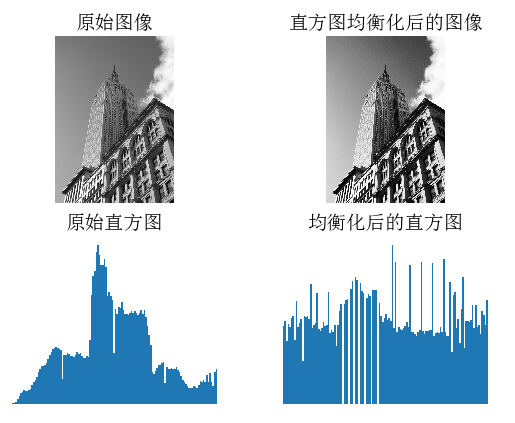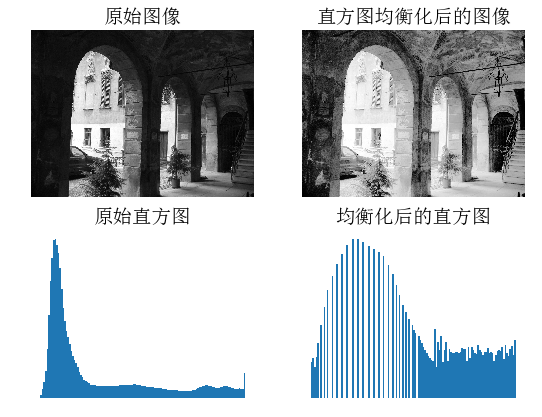1.3.5 图像平均

对图像取平均是一种图像降噪的简单方法，经常用于产生艺术效果。假设所有的图像具有相同的尺寸，我们可以对图像相同位置的像素相加取平均，下面是一个演示对图像取平均的例子：

def compute_average(imlist):
""" 计算图像列表的平均图像"""

# 打开第一幅图像，将其存储在浮点型数组中
averageim = array(Image.open(imlist), 'f')

for imname in imlist[1:]:
try:
averageim += array(Image.open(imname))
except:
print imname + '...skipped'
averageim /= len(imlist)

# 返回uint8 类型的平均图像
return array(averageim, 'uint8')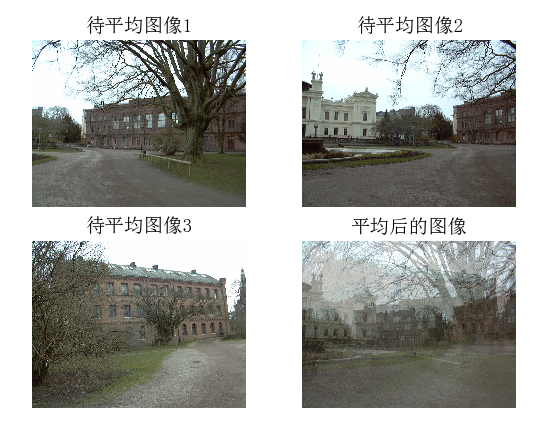注意：有可能因为某些图像打不开而导致平均的结果只是某一幅自身或某两幅图像的平均

1.3.5 对图像进行主成分分析

PCA（Principal Component Analysis，主成分分析）是一个非常有用的降维技巧。它可以在使用尽可能少维数的前提下，尽量多地保持训练数据的信息，在此意义上是一个最佳技巧。即使是一幅 100×100 像素的小灰度图像，也有 10 000 维，可以看成 10 000 维空间中的一个点。一兆像素的图像具有百万维。由于图像具有很高的维数，在许多计算机视觉应用中，我们经常使用降维操作。PCA 产生的投影矩阵可以被视为将原始坐标变换到现有的坐标系，坐标系中的各个坐标按照重要性递减排列。

为了对图像数据进行 PCA 变换，图像需要转换成一维向量表示。我们可以使用 NumPy 类库中的flatten() 方法进行变换。

将变平的图像堆积起来，我们可以得到一个矩阵，矩阵的一行表示一幅图像。在计算主方向之前，所有的行图像按照平均图像进行了中心化。我们通常使用 SVD（Singular Value Decomposition，奇异值分解）方法来计算主成分；但当矩阵的维数很大时，SVD 的计算非常慢，所以此时通常不使用 SVD 分解。

下面就是 PCA 操作的代码：

from PIL import Image
from numpy import *

def pca(X):
""" 主成分分析：
输入：矩阵X ，其中该矩阵中存储训练数据，每一行为一条训练数据
返回：投影矩阵（按照维度的重要性排序）、方差和均值"""

# 获取维数
num_data,dim = X.shape

# 数据中心化
mean_X = X.mean(axis=0)
X = X - mean_X

if dim>num_data:
# PCA- 使用紧致技巧
M = dot(X,X.T) # 协方差矩阵
e,EV = linalg.eigh(M) # 特征值和特征向量
tmp = dot(X.T,EV).T # 这就是紧致技巧
V = tmp[::-1] # 由于最后的特征向量是我们所需要的，所以需要将其逆转
S = sqrt(e)[::-1] # 由于特征值是按照递增顺序排列的，所以需要将其逆转
for i in range(V.shape):
V[:,i] /= S
else:
# PCA- 使用SVD 方法
U,S,V = linalg.svd(X)
V = V[:num_data] # 仅仅返回前nun_data 维的数据才合理

# 返回投影矩阵、方差和均值
return V,S,mean_X

该函数首先通过减去每一维的均值将数据中心化，然后计算协方差矩阵对应最大特征值的特征向量，此时可以使用简明的技巧或者 SVD 分解。这里我们使用了 range() 函数，该函数的输入参数为一个整数 n，函数返回整数 0…(n-1) 的一个列表。你也可以使用 arange() 函数来返回一个数组，或者使用 xrange() 函数返回一个产生器（可能会提升速度）。我们在本书中贯穿使用range() 函数。

如果数据个数小于向量的维数，我们不用 SVD 分解，而是计算维数更小的协方差矩阵 XXT 的特征向量。通过仅计算对应前 k（k 是降维后的维数）最大特征值的特征向量，可以使上面的 PCA 操作更快。由于篇幅所限，有兴趣的读者可以自行探索。矩阵 V 的每行向量都是正交的，并且包含了训练数据方差依次减少的坐标方向。

我们接下来对字体图像进行 PCA 变换。fontimages.zip 文件包含采用不同字体的字符 a 的缩略图。所有的 2359 种字体可以免费下载 2。假定这些图像的名称保存在列表 imlist 中，跟之前的代码一起保存传在 pca.py 文件中，我们可以使用下面的脚本计算图像的主成分：

import pickle
from PIL import Image
from numpy import *
from pylab import *
from PCV.tools import imtools,pca

# Uses sparse pca codepath

# 获取图像列表和尺寸
imlist=imtools.get_imlist('E:/python/Python Computer Vision/Image data/fontimages/a_thumbs')
# open ont image to get the size
im=array(Image.open(imlist))
# get the size of the images
m,n=im.shape[:2]
# get the number of images
imnbr=len(imlist)
print("The number of images is %d" % imnbr)

# create matrix to store all flattened images
immatrix = array([array(Image.open(imname)).flatten() for imname in imlist],'f')

# PCA降维
V,S,immean=pca.pca(immatrix)

# 保存均值和主成分
#f = open('../ch01/font_pca_modes.pkl', 'wb')
#pickle.dump(immean,f)
#pickle.dump(V,f)
#f.close()

# Show the images (mean and 7 first modes)
# This gives figure 1-8 (p15) in the book.

figure()
gray()
subplot(241)
axis('off')
imshow(immean.reshape(m,n))
for i in range(7):
subplot(2,4,i+2)
imshow(V[i].reshape(m,n))
axis('off')

show()



注意，这些图像在拉成一维表示后，必须用reshape()函数将它重新转换回来。运行上面代码，可得原书P15 Figure1-8中的结果，即：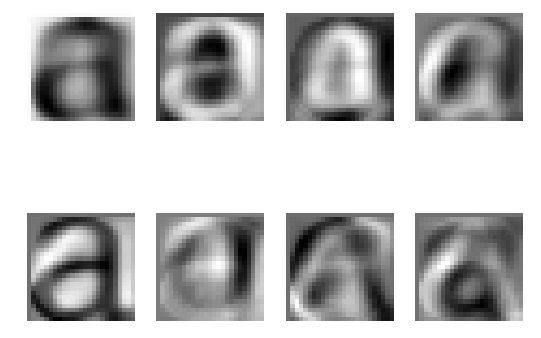1.3.6 Pickle模块

如果想要保存一些结果或者数据以方便后续使用，Python 中的 pickle 模块非常有用。pickle模块可以接受几乎所有的 Python 对象，并且将其转换成字符串表示，该过程叫做封装（pickling）。从字符串表示中重构该对象，称为拆封（unpickling）。这些字符串表示可以方便地存储和传输。

我们来看一个例子。假设想要保存上一节字体图像的平均图像和主成分，可以这样来完成：

# 保存均值和主成分数据
f = open('font_pca_modes.pkl','wb')
pickle.dump(immean,f)
pickle.dump(V,f)
f.close()

在上述例子中，许多对象可以保存到同一个文件中。pickle 模块中有很多不同的协议可以生成 .pkl 文件；如果不确定的话，最好以二进制文件的形式读取和写入。在其他 Python 会话中载入数据，只需要如下使用 load() 方法：

# 载入均值和主成分数据
f = open('font_pca_modes.pkl','rb')
f.close()

注意，载入对象的顺序必须和先前保存的一样。Python 中有个用 C 语言写的优化版本，叫做cpickle 模块，该模块和标准 pickle 模块完全兼容。关于 pickle 模块的更多内容，参见pickle 模块文档页 http://docs.python.org/library/pickle.html。

在本书接下来的章节中，我们将使用 with 语句处理文件的读写操作。这是 Python 2.5 引入的思想，可以自动打开和关闭文件（即使在文件打开时发生错误）。下面的例子使用 with() 来实现保存和载入操作：

# 打开文件并保存
with open('font_pca_modes.pkl', 'wb') as f:
pickle.dump(immean,f)
pickle.dump(V,f)

和

# 打开文件并载入
with open('font_pca_modes.pkl', 'rb') as f:
V = pickle.load(f)

上面的例子乍看起来可能很奇怪，但 with() 确实是个很有用的思想。如果你不喜欢它，可以使用之前的 open 和 close 函数。

作为 pickle 的一种替代方式，NumPy 具有读写文本文件的简单函数。如果数据中不包含复杂的数据结构，比如在一幅图像上点击的点列表，NumPy 的读写函数会很有用。保存一个数组 x 到文件中，可以使用：

savetxt('test.txt',x,'%i')

最后一个参数表示应该使用整数格式。类似地，读取可以使用：

x = loadtxt('test.txt')

可以从在线文档了解更多

最后，NumPy 有专门用于保存和载入数组的函数，在线文档中可以查看关于 save()和 load() 的更多内容。

1.4 SciPy

SciPy（http://scipy.org/） 是建立在 NumPy 基础上，用于数值运算的开源工具包。SciPy 提供很多高效的操作，可以实现数值积分、优化、统计、信号处理，以及对我们来说最重要的图像处理功能。

1.4.1 图像模糊

图像的高斯模糊是非常经典的图像卷积例子。本质上，图像模糊就是将（灰度）图像I $I$$I$ 和一个高斯核进行卷积操作：
I δ =I∗G δ  ${I}_{\delta }=I\ast {G}_{\delta }$$I_\delta=I* G_\delta$

其中，*表示卷积，G δ  ${G}_{\delta }$$G_\delta$表示标准差为δ $\delta$$\delta$的卷积核

滤波操作模块——scipy.ndimage.filters

该模块可以使用快速一维分离的方式来计算卷积，使用方式如下：

from PIL import Image
from numpy import *
from pylab import *
from scipy.ndimage import filters

# 添加中文字体支持
from matplotlib.font_manager import FontProperties
font=FontProperties(fname=r"c:\windows\fonts\SimSun.ttc",size=14)

im=array(Image.open('E:/python/Python Computer Vision/Image data/empire.jpg').convert('L'))

figure()
gray()
axis('off')
subplot(141)
axis('off')
title(u'原图',fontproperties=font)
imshow(im)

for bi,blur in enumerate([2,5,10]):
im2=zeros(im.shape)
im2=filters.gaussian_filter(im,blur)
im2=np.uint8(im2)
imNum=str(blur)
subplot(1,4,2+bi)
axis('off')
title(u'标准差为'+imNum,fontproperties=font)
imshow(im2)

#如果是彩色图像，则分别对三个通道进行模糊
#for bi, blur in enumerate([2, 5, 10]):
#  im2 = zeros(im.shape)
#  for i in range(3):
#    im2[:, :, i] = filters.gaussian_filter(im[:, :, i], blur)
#  im2 = np.uint8(im2)
#  subplot(1, 4,  2 + bi)
#  axis('off')
#  imshow(im2)

show()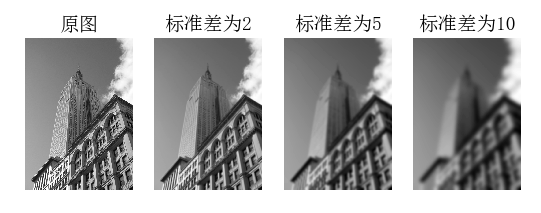上面第一幅图为待模糊图像，第二幅用高斯标准差为2进行模糊，第三幅用高斯标准差为5进行模糊，最后一幅用高斯标准差为10进行模糊。关于该模块的使用以及参数选择的更多细节，可以参阅SciPy scipy.ndimage文档

1.4.2 图像导数

在很多应用中图像强度的变化情况是非常重要的信息。强度的变化可以用灰度图像 I $I$$I$（对于彩色图像，通常对每个颜色通道分别计算导数）的 x $x$$x$ 和 y $y$$y$方向导数 I x  ${I}_{x}$$I_x$ 和I y  ${I}_{y}$$I_y$ 进行描述。

图像的梯度向量为∇I=[I x ,I y ] T  $\nabla I=\left[{I}_{x},{I}_{y}{\right]}^{T}$$∇I = [I_x, I_y]^T$，描述图像在每个像素点上强度变化最大的方向。
梯度有两个重要的属性：
梯度的大小：
|∇I|=I 2 x +I 2 y  − − − − − −  √  $|\nabla I|=\sqrt{{I}_{x}^{2}+{I}_{y}^{2}}$$|∇I| = \sqrt {I_x^2+I_y^2}$
梯度的方向：
α=arctan2(I x ,I y ) $\alpha =arctan2\left({I}_{x},{I}_{y}\right)$$\alpha=arctan2(I_x, I_y)$

NumPy中的arctan2()函数返回弧度表示的有符号角度，角度的变化区间为[−π,π] $\left[-\pi ,\pi \right]$$[-\pi,\pi]$

我们可以用离散近似的方式来计算图像的导数。图像导数大多数可以通过卷积简单地实现：
I x =I∗D x  ${I}_{x}=I\ast {D}_{x}$$I_x=I*D_x$，I y =I∗D y  ${I}_{y}=I\ast {D}_{y}$$I_y=I*D_y$
对于，通常选择prewitt滤波器或sobel滤波器
这些导数滤波器可以使用scipy.ndimage.filters模块的标准卷积操作来简单实现

from PIL import Image
from pylab import *
from scipy.ndimage import  filters
import numpy

# 添加中文字体支持
from matplotlib.font_manager import FontProperties
font=FontProperties(fname=r"c:\windows\fonts\SimSun.ttc",size=14)

im=array(Image.open('E:/python/Python Computer Vision/Image data/empire.jpg').convert('L'))
gray()

subplot(141)
axis('off')
title(u'(a)原图',fontproperties=font)
imshow(im)

# sobel derivative filters
imx=zeros(im.shape)
filters.sobel(im,1,imx)
subplot(142)
axis('off')
title(u'(b)x方向差分',fontproperties=font)
imshow(imx)

imy=zeros(im.shape)
filters.sobel(im,0,imy)
subplot(143)
axis('off')
title(u'(c)y方向差分',fontproperties=font)
imshow(imy)

mag=255-numpy.sqrt(imx**2+imy**2)
subplot(144)
title(u'(d)梯度幅值',fontproperties=font)
axis('off')
imshow(mag)

show()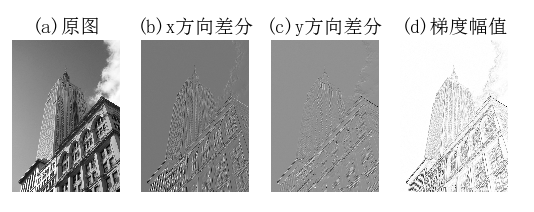高斯差分：

from PIL import Image
from pylab import *
from scipy.ndimage import filters
import numpy

# 添加中文字体支持
#from matplotlib.font_manager import FontProperties
#font = FontProperties(fname=r"c:\windows\fonts\SimSun.ttc", size=14)

def imx(im, sigma):
imgx = zeros(im.shape)
filters.gaussian_filter(im, sigma, (0, 1), imgx)
return imgx

def imy(im, sigma):
imgy = zeros(im.shape)
filters.gaussian_filter(im, sigma, (1, 0), imgy)
return imgy

def mag(im, sigma):
#mag = numpy.sqrt(imgx**2 + imgy**2)
imgmag = 255 - numpy.sqrt(imgx ** 2 + imgy ** 2)
return imgmag

im = array(Image.open('E:/python/Python Computer Vision/Image data/empire.jpg').convert('L'))
figure()
gray()

sigma = [2, 5, 10]

for i in  sigma:
subplot(3, 4, 4*(sigma.index(i))+1)
axis('off')
imshow(im)
imgx=imx(im, i)
subplot(3, 4, 4*(sigma.index(i))+2)
axis('off')
imshow(imgx)
imgy=imy(im, i)
subplot(3, 4, 4*(sigma.index(i))+3)
axis('off')
imshow(imgy)
imgmag=mag(im, i)
subplot(3, 4, 4*(sigma.index(i))+4)
axis('off')
imshow(imgmag)

show()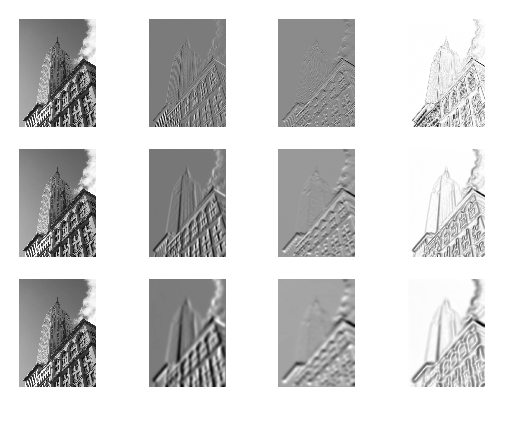1.4.3 形态学：对象计数

形态学（或数学形态学）是度量和分析基本形状的图像处理方法的基本框架与集合。形态学通常用于处理二值图像，但是也能够用于灰度图像。二值图像是指图像的每个像素只能取两个值，通常是 0 和 1。二值图像通常是，在计算物体的数目，或者度量其大小时，对一幅图像进行阈值化后的结果。可以从 http://en.wikipedia.org/wiki/Mathematical_morphology 大体了解形态学及其处理图像的方式。

scipy.ndimage 中的 morphology 模块可以实现形态学操作
scipy.ndimage 中的measurements 模块来实现二值图像的计数和度量功能

下面通过一个简单的例子介绍如何使用它们：

from scipy.ndimage import measurements,morphology

# 载入图像，然后使用阈值化操作，以保证处理的图像为二值图像
im = array(Image.open('houses.png').convert('L'))
im = 1*(im<128)

labels, nbr_objects = measurements.label(im)
print "Number of objects:", nbr_objects

上面的脚本首先载入该图像，通过阈值化方式来确保该图像是二值图像。通过和 1 相乘，脚本将布尔数组转换成二进制表示。
然后，我们使用 label() 函数寻找单个的物体，并且按照它们属于哪个对象将整数标签给像素赋值。
图 1-12b 是 labels 数组的图像。图像的灰度值表示对象的标签。可以看到，在一些对象之间有一些小的连接。进行二进制开（binary open）操作，我们可以将其移除：

# 形态学开操作更好地分离各个对象
im_open = morphology.binary_opening(im,ones((9,5)),iterations=2)

labels_open, nbr_objects_open = measurements.label(im_open)
print "Number of objects:", nbr_objects_open

binary_opening() 函数的第二个参数指定一个数组结构元素。

该数组表示以一个像素为中心时，使用哪些相邻像素。
在这种情况下，我们在 y 方向上使用 9 个像素（上面 4 个像素、像素本身、下面 4 个像素），在 x 方向上使用 5 个像素。你可以指定任意数组为结构元素，数组中的非零元素决定使用哪些相邻像素。
参数 iterations 决定执行该操作的次数。你可以尝试使用不同的迭代次数 iterations 值，看一下对象的数目如何变化。
可以在图 1-12c 与图 1-12d 中查看经过开操作后的图像，以及相应的标签图像。
binary_closing() 函数实现相反的操作。

我们将该函数和在 morphology 和 measurements 模块中的其他函数的用法留作练习。你可以从 scipy.ndimage 模块文档  中了解关于这些函数的更多知识。

from PIL import Image
from numpy import *
from scipy.ndimage import measurements, morphology
from pylab import *

"""   This is the morphology counting objects example in Section 1.4.  """

# 添加中文字体支持
from matplotlib.font_manager import FontProperties
font = FontProperties(fname=r"c:\windows\fonts\SimSun.ttc", size=14)

# load image and threshold to make sure it is binary
figure()
gray()
im = array(Image.open('E:/python/Python Computer Vision/Image data/houses.png').convert('L'))
subplot(221)
imshow(im)
axis('off')
title(u'原图', fontproperties=font)
im = (im < 128)

labels, nbr_objects = measurements.label(im)
print ("Number of objects:", nbr_objects)
subplot(222)
imshow(labels)
axis('off')
title(u'标记后的图', fontproperties=font)

# morphology - opening to separate objects better
im_open = morphology.binary_opening(im, ones((9, 5)), iterations=2)
subplot(223)
imshow(im_open)
axis('off')
title(u'开运算后的图像', fontproperties=font)

labels_open, nbr_objects_open = measurements.label(im_open)
print ("Number of objects:", nbr_objects_open)
subplot(224)
imshow(labels_open)
axis('off')
title(u'开运算后进行标记后的图像', fontproperties=font)

show()

输出：

Number of objects: 45
Number of objects: 48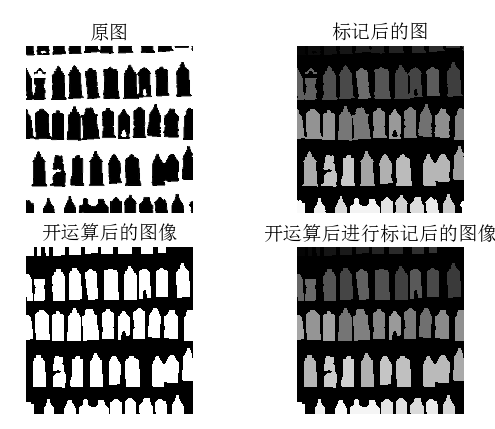1.4.4 有用的SciPy模块

SciPy 中包含一些用于输入和输出的实用模块。下面介绍其中两个模块：io 和 misc

1.读写.mat文件

如果你有一些数据，或者在网上下载到一些有趣的数据集，这些数据以 Matlab 的 .mat 文件格式存储，那么可以使用 scipy.io 模块进行读取。

data = scipy.io.loadmat('test.mat')

上面代码中，data 对象包含一个字典，字典中的键对应于保存在原始 .mat 文件中的变量名。由于这些变量是数组格式的，因此可以很方便地保存到 .mat 文件中。你仅需创建一个字典（其中要包含你想要保存的所有变量），然后使用 savemat() 函数：

data = {}
data['x'] = x
scipy.io.savemat('test.mat',data)

因为上面的脚本保存的是数组 x，所以当读入到 Matlab 中时，变量的名字仍为 x。关于scipy.io 模块的更多内容，请参见在线文档。

2.以图像形式保存数组

因为我们需要对图像进行操作，并且需要使用数组对象来做运算，所以将数组直接保存为图像文件 4 非常有用。本书中的很多图像都是这样的创建的。

imsave() 函数：从 scipy.misc 模块中载入。要将数组 im 保存到文件中，可以使用下面的命令：

from scipy.misc import imsave
imsave('test.jpg',im)

scipy.misc 模块同样包含了著名的 Lena 测试图像：

lena = scipy.misc.lena()

该脚本返回一个 512×512 的灰度图像数组

所有 Pylab 图均可保存为多种图像格式，方法是点击图像窗口中的“保存”按钮。

1.5 高级示例：图像去噪

我们通过一个非常实用的例子——图像的去噪——来结束本章。图像去噪是在去除图像噪声的同时，尽可能地保留图像细节和结构的处理技术。我们这里使用 ROF（Rudin-Osher-Fatemi）去噪模型。该模型最早出现在文献  中。图像去噪对于很多应用来说都非常重要；这些应用范围很广，小到让你的假期照片看起来更漂亮，大到提高卫星图像的质量。ROF 模型具有很好的性质：使处理后的图像更平滑，同时保持图像边缘和结构信息。

ROF 模型的数学基础和处理技巧非常高深，不在本书讲述范围之内。在讲述如何基于 Chambolle 提出的算法  实现 ROF 求解器之前，本书首先简要介绍一下 ROF 模型。

降噪综合示例：

from pylab import *
from numpy import *
from numpy import random
from scipy.ndimage import filters
from scipy.misc import imsave
from PCV.tools import rof

""" This is the de-noising example using ROF in Section 1.5. """

# 添加中文字体支持
from matplotlib.font_manager import FontProperties
font = FontProperties(fname=r"c:\windows\fonts\SimSun.ttc", size=14)

# create synthetic image with noise
im = zeros((500,500))
im[100:400,100:400] = 128
im[200:300,200:300] = 255
im = im + 30*random.standard_normal((500,500))

U,T = rof.denoise(im,im)
G = filters.gaussian_filter(im,10)

# save the result
#imsave('synth_original.pdf',im)
#imsave('synth_rof.pdf',U)
#imsave('synth_gaussian.pdf',G)

# plot
figure()
gray()

subplot(1,3,1)
imshow(im)
#axis('equal')
axis('off')
title(u'原噪声图像', fontproperties=font)

subplot(1,3,2)
imshow(G)
#axis('equal')
axis('off')
title(u'高斯模糊后的图像', fontproperties=font)

subplot(1,3,3)
imshow(U)
#axis('equal')
axis('off')
title(u'ROF降噪后的图像', fontproperties=font)

show()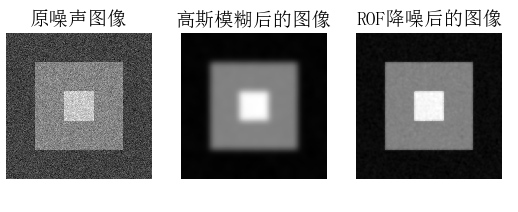其中第一幅图示原噪声图像，中间一幅图示用标准差为10进行高斯模糊后的结果，最右边一幅图是用ROF降噪后的图像。上面原噪声图像是模拟出来的图像，现在我们在真实的图像上进行测试：

from PIL import Image
from pylab import *
from numpy import *
from numpy import random
from scipy.ndimage import filters
from scipy.misc import imsave
from PCV.tools import rof

""" This is the de-noising example using ROF in Section 1.5. """

# 添加中文字体支持
from matplotlib.font_manager import FontProperties
font = FontProperties(fname=r"c:\windows\fonts\SimSun.ttc", size=14)

im = array(Image.open('E:/python/Python Computer Vision/Image data/empire.jpg').convert('L'))

U,T = rof.denoise(im,im)
G = filters.gaussian_filter(im,10)

# save the result
#imsave('synth_original.pdf',im)
#imsave('synth_rof.pdf',U)
#imsave('synth_gaussian.pdf',G)

# plot
figure()
gray()

subplot(1,3,1)
imshow(im)
#axis('equal')
axis('off')
title(u'原噪声图像', fontproperties=font)

subplot(1,3,2)
imshow(G)
#axis('equal')
axis('off')
title(u'高斯模糊后的图像', fontproperties=font)

subplot(1,3,3)
imshow(U)
#axis('equal')
axis('off')
title(u'ROF降噪后的图像', fontproperties=font)

show()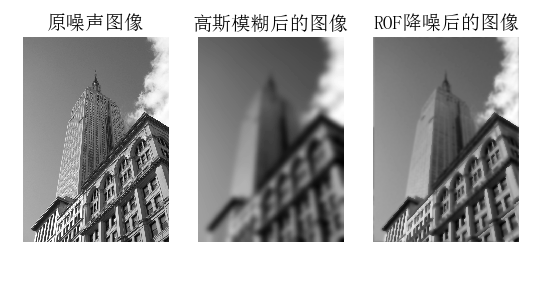ROF降噪能够保持边缘和图像结构

Number of objects: 45
Number of objects: 48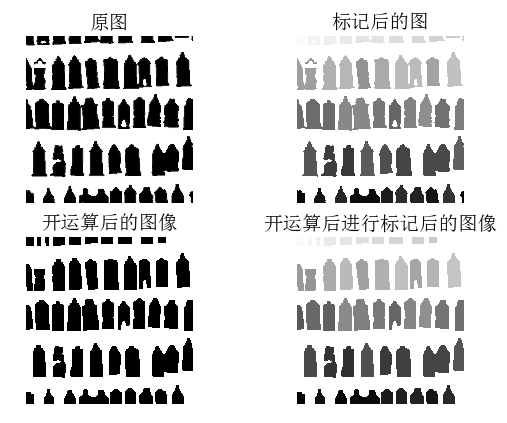展开全文python计算机视觉
• 经常需要使用 Python 对图像做处理，那就免不了使用一些图像处理库，特地写了这篇博客记录一下常用图像处理库。

经常需要使用 Python 对图像做处理，那就免不了使用一些图像处理库，特地写了这篇博客记录一下常用的图像处理库。

目录

OpenCV 速查表
PIL 速查表
Matplotlib 速查表

1. OpenCV 速查表

Open Source Computer Vision, 其更广为人知的名字是OpenCV，是 Intel 开源计算机视觉库，由一系列 C 函数和少量 C++ 类构成，实现了图像处理和计算机视觉方面的很多通用算法。
OpenCV 使用 C/C++ 开发，同时也提供了 Python、Java、MATLAB 等其他语言的接口。本文主要是对 OpenCV-Python 的接口进行描述。
此处为相应的文档链接：[英文] [中文]

由 OpenCV 支持的主要格式有：

Windows bitmaps（*.bmp、*dib）；
Portable image formats（.pbm、.pgm、*.ppm）；
Sun rasters（.sr、.ras）；

需要辅助库的格式有：

JPEG（.jpeg、.jpg、*.jpe）；
JPEG 2000（*.jp2）；
Portable Network Graphics（*.png）；
TIFF（.tiff、.tif）；
WebP（*.webp）；

-读取图像并显示图像
import cv2
# 读取图像，默认读入彩色图像
# 除了上面这三个标志，你可以简单地传递整数 1，0，-1。
img = cv2.imread('example.jpg') # 当该图像不存在时，返回 None
# 显示图像
cv2.namedWindow('example',cv2.WINDOW_NORMAL) # 创建窗口
cv2.imshow('example',example) # 在指定窗口中显示图像
cv2.waitKey(0) # 等待键盘输入，若传入参数为0，则程序将无限制等待用户的案件事件，否则，程序等待所传入参数对应的毫秒时间后，跳出循环。
# 如果程序想响应某个按键，可这样写 ：
# if waitKey(0)==Keyvalue：
#     pass
cv2.destroyAllWindows() # 删除所有建立的窗口，若需删除指定窗口，则使用cv2.destroyWindow()，并传入指定窗口的名称。

-保存图像
import cv2
# 读取图像
# 保存图像
# 还可以指定保存图像的品质或压缩率
# 对于 jpg 图像，cv2.imwrite(path,img,[cv2.IMWRITE_JPEG_QUALITY,90])，参数范围为 0~100
# 对于 png 图像，cv2.imwrite(path,img,[cv2.IMWRITE_PNG_COMPRESSION,5])，参数范围为 0~9
cv.imwrite('example.png')

-转换图像格式
import cv2
# 由 RGB 转为 灰度图
img = cv2.cvtColor(img,cv2.COLOR_BGR2GRAY)
# 由 RGB 转为 HSV
img = cv2.cvtColor(img,cv2.COLOR_BGR2HSV)

-图像坐标轴
注意：在 OpenCV 中，RGB 图像的通道顺序为 BGR。
OpenCV 所读进来的图像本身就是一个 np.ndarray 实例，其维度为 (height,width,channels)，其原点坐标位于图像的左上角。
-常用方法
import cv2
# 读取图像
# 图像缩放
# cv2 的图像维度一般以 (height,width,channel)存在，但 resize 不同，以(width,height,channel)存在，且默认采用双线性插值。
# 可选参数 nninterpolation 的取值范围：
# cv2.INTER_NEAREST	最近邻插值
# cv2.INTER_LINEAR	双线性插值（默认设置）
# cv2.INTER_AREA	使用像素区域关系进行重采样。 它可能是图像抽取的首选方法，因为它会产生无云纹理的结果。 但是当图像缩放时，它类似于 INTER_NEAREST方法。
# cv2.INTER_CUBIC	4x4像素邻域的双三次插值
# cv2.INTER_LANCZOS4	8x8像素邻域的Lanczos插值
img = cv2.resize(img,(width,height))
# 通道分离
b,g,r = cv2.split(img)
# 合并通道
img = cv2.merge((b,g,r))
# 复制图像
img = img.copy()
# 获取ROI
ROI = img[top:low,left:right]
# 由 PIL 格式转化为 OpenCV 格式
img = cv2.cvtColor(numpy.asarray(image),cv2.COLOR_RGB2BGR)


2. PIL 速查表

Python Imaging Library ，或者叫 PIL，简略来说， 是Python图像操作的核心库。
不幸的是，它的开发陷入了停滞，最后一次更新是2009年，只支持到 Python 2.7。
不过，目前仍存在一个活跃的 PIL 开发分支，叫做 Pillow。它很容易安装，运行在虽有的主流操作系统上，而且支持Python 3.x。
此处为相应的文档链接：[英文] [中文]

读取图像，查看图像信息并显示图像

from PIL import Image
# 读取图像
# Image.open() 返回 Image 类的实例，不需要指定图像格式，由文件内容自动确定图像格式。
img = Image.open('example.jpg')
# 显示图像
img.show()
# Image 类有5个属性，分别如下所示：
# format : 返回图像的格式(PNG,JPG,None等，。如果图像不是从文件读取的，它的值就是 None。
# mode : 返回图像的模式，常用模式有 L (luminance) 表示灰度图像, RGB 表示真彩色图像, CMYK 表示出版图像。
# size : 返回 (width, height)，宽度和高度，单位都是px。
# palette : 仅当 mode 为 P 时有效，返回 ImagePalette 实例。
# info : 以字典的形式返回实例的信息。


保存图像

from PIL import Image
# 读取图像
img = Image.open('example.jpg')
# 保存图像
# 调用 Image 类的函数 save，save(filename,format) 函数以特定的图像格式保存图像。
# 若不指定图像格式，save() 方法会根据文件扩展名来选择相应的图像格式。
img.save('OtherExample.jpg')


转换图像模式

from PIL import Image
img = Image.open('example.jpg')
# 由 RGB 模式转为 L 模式，即将彩色图像转为灰度图像
img = img.convert('L')


图像与张量的转换

import numpy as np
from PIL import Image
# 读取图像
img = Image.open('example.jpg')
# 将 Image 实例转换为 np.ndarray 实例
img = np.array(img)
# 将 np.ndarray 实例转换为 Image 实例
img = Image.fromarray(img)


图像坐标轴
由于 PIL 读取图像后并不是直接以 np.ndarray 的形式存在的，而是以 Image 实例的形式所存在。
所以要想探究图像的坐标轴，我们得先进行图像与张量得转换。
查阅资料后，可以得知：
图片坐标和数组坐标是相反的，坐标原点位于左上角。
图片坐标第一维是横向向右，第二维纵向向下。
但是数组坐标第一维是纵向向下，第二维横向向右。

import numpy as np
from PIL import Image
# 读取图像
img = Image.open('example.jpg')
print(img.size) # 输出：(400,200)
# 将 Image 实例转换为 np.ndarray 实例
img = np.array(img)
print(img.shape)# 输出：(200,400,3)


常用方法

from PIL import Image
# 读取图像
img = Image.open('example.jpg')
# 图像缩放
img = img.resize((width, height))
# 带 ANTIALIAS 滤镜缩放结果的图像缩放
img = img.resize((width, height),Image.ANTIALIAS)
# 通道分离
r, g, b = img.split()
# 合并通道
img = Image.merge("RGB", (b, g, r))
# 复制图像
img = img.copy()
# 获取 ROI
ROI = img.crop((left,upper,right,lower))
# 由 OpenCV 格式转化为 PIL 格式
image = Image.fromarray(cv2.cvtColor(img,cv2.COLOR_BGR2RGB))


3. Matplotlib 速查表

Matplotlib 应该是 Python 2D-绘图领域使用最广泛的套件，它能让使用者很轻松地将数据图形化，并且提供多样化的输出格式。
Matplotlib 的功能和 Matlab 中的画图的功能十分类似，但是 Matlab 进行画图相对来说比较复杂，所以使用 Python 中的 Matplotlib 来画图比较方便。
此处为相应的文档链接：[英文] [中文]

读取图像并展示图像
import matplotlib.pyplot as plt # plt 用于显示图片
import matplotlib.image as mpimg # mpimg 用于读取图片

# 读取图像

# 展示图像
plt.figure("Image") # 设置窗口名称，窗口大小可通过可选参数 figsize 进行设置
plt.imshow(img)　   # 绘制图像，若图像为灰度图，应这样写：plt.imshow(img,cmap='gray')
plt.axis('on') 	    # 显示坐标轴，若不想展示坐标轴，应这样写：plt.axis('off')
plt.title('image')  # 设置图像名称
plt.show()　　　　　　# 展示图像

img = Image.open(os.path.join('images', '2007_000648' + '.jpg'))
gray = img.convert('L')
r,g,b = img.split()
img_merged = Image.merge('RGB', (r, g, b))

# 同时展示多副图像
plt.figure("Other Image")
plt.suptitle('Multi_Image')
plt.subplot(2,2,1), plt.title('1'), plt.imshow(img),plt.axis('off')
plt.subplot(2,2,2), plt.title('2'), plt.imshow(img),plt.axis('off')
plt.subplot(2,2,3), plt.title('3'), plt.imshow(img),plt.axis('off')
plt.subplot(2,2,4), plt.title('4'), plt.imshow(img),plt.axis('off')
plt.show()

# 保存图像
plt.savefig("example.jpg")

常用函数
import matplotlib.pyplot as plt # plt 用于显示图片
import matplotlib.image as mpimg # mpimg 用于读取图片

# 绘制函数

# 绘制单个函数：
plot([x], y, [format1])
# 绘制多个函数：
plot([x], y, [format1], [x2], y2, [format2], ..., [xn], [yn], [formatn])
# 绘制多个函数并对每个函数进行区分：
plot([x], y, [format1], [label1], [x2], y2, [format2], [label2], ..., [xn], [yn], [formatn], [labeln])
# 可选参数 [format] 是一个字符串来定义图的基本属性如：颜色（color），点型（marker），线型（linestyle），
# 具体形式 format = '[color][marker][line]'
# format 接收的是每个属性的单个字母缩写，例如：
# plot(x, y, 'bo-')  # 蓝色圆点实线

# 绘制直方图
plt.bar(x, y)
# plt.bar(left, height, width=0.8, bottom=None, **kwargs)
# left，height，width，bottom这四个参数确定了柱体的位置和大小。
# 默认情况下，left为柱体的居中位置（可以通过align参数来改变left值的含义），即：
# (left - width / 2, bottom)为左下角位置
# (left + width / 2, bottom + height)为右上角位置

参考资料：

图像处理
Python各类图像库的图片读写方式总结
OpenCV-Python中文教程
OpenCV图像坐标系与行列宽高的关系
python matplotlib中常用函数
Python图表绘制：matplotlib绘图库入门
Python之Matplotlib库常用函数大全（含注释）
python 读取并显示图片的两种方法
Pyplot 教程
matplotlib绘图——柱状图

如果你看到了这篇文章的最后，并且觉得有帮助的话，麻烦你花几秒钟时间点个赞，或者受累在评论中指出我的错误。谢谢！
作者信息：
知乎：没头脑
LeetCode：Tao Pu
CSDN：Code_Mart
Github：Bojack-want-drink


展开全文python 图像处理库
• 读取图像 imread(图像路径[, 读取图像的参数]) img = cv2.imread("/Users/zhaozhengwei/Desktop/photo/lbj.png") 显示图像 imshow(“窗口名”, 读取到的图像) cv2.imshow("demo", img) 显示等待 waitKey([,...


读取图像
img = cv2.imread("/Users/zhaozhengwei/Desktop/photo/lbj.png")


显示图像
imshow(“窗口名”, 读取到的图像)
cv2.imshow("demo", img)


显示等待
waitKey([,等待参数])
cv2.waitKey(0) #无限等待


保存图像
imwrite(要保存的路径,要保存的图像)
cv2.imwrite("/Users/zhaozhengwei/Desktop/photo/lbj.png",img)


关闭窗口
destroyWindow(“窗口名”) # 关闭指定窗口
destroyAllWindows() # 关闭所有窗口
cv2.destroyAllWindows()


像素处理
获取图像参数：图像(位置参数)
img[x,y] #获取图像一个像素点的值，如果为单通道则返回一个值，多通道返回这个点所有通道的值(列表形式)
blue = img[x,y,n] #获取多通道图像中一个点的一个指定通道n的值
img[x,y] =255 # 给指定像素点赋值
p = img[100,100]
blue = img[100,100,0]
img[100,100] =255


获取图像的属性
img.shape #返回图像的行数、列数、通道数
img.size # 返回图像的大小，行数列数通道数
img.dtype # 返回图像的数据类型，如uinti8等

获取图像区域
frame = img.[200：400，200：400] #获取 200行到400行， 200行到400列之间的图像
img.[200：400，200：400] = frame #将图像复制到200行到400行， 200行到400列之间的区域


图像通道拆分合并
b, g, r = cv2.split(img) # 将多通道拆分为单通道
cv2.merge([b, g, r]) # 将多个单通道合并为多通道


图像相加
img = img1 + img2 #直接相加，如果像素相加后大于255则对255取模


图像融合
img = cv2.addWeighted(img1, a, img2, b, 0) #a, b 分别为两张图像融合时的权重，0 为增加的亮度值。


图像转换
img = cv2.cvtColor(img, cv2.COLOR_BGR2GRAY) #将图像从bgr格式转化为gray


图像缩放
img = cv2.resize(img, (100, 100)) #将图像缩放为100*100 大小
img = cv2.resize(img, None, fx=0.5, fy=0.7)) #将图像在x轴方向缩小为0.5，y轴方向缩小0.7


图像反转
img = cv2.flip(img, filpCode) #filpCode=0 为上下翻转，filpCode>0 为左右翻转，filpCode < 0为先上下翻转再左右翻转。


图像阈值分割
rst, img = (img, thresh, maxval, type)
img为图像，thresh为阈值，maxval为最大值， type为类型
rst, img = (img, 127, 255, cv2.THRESH_BINARY) # 二值阈值化：像素值小于等于127则为0，大于127则为255
rst, img = (img, 127, 255, cv2.THRESH_BINARY_INV) # 反二值阈值化：和上面相反，像素值小于等于127则为255，大于127则为0
rst, img = (img, 127, 255, cv2.THRESH_TRUNC) # 截断阈值化：像素值小于127则为原值，大于127则为127
rst, img = (img, 127, 255, cv2.THRESH_TOZERO) # 阈值化为0：像素值小于等于127则为0，大于127则不变
rst, img = (img, 127, 255, cv2.THRESH_TOZERO_INV) # 反阈值化为0：和上面相反，像素值小于等于127则为原值，大于127则为0


待续。。。


展开全文python opencv
• Python环境下的图像处理库种类较多，无论是精度，速度等方面都有不同的差异，这里予以总结。 Python环境下，相关的包有： Numpy：主要作为图像数据的中转 Skimage：速度较慢，主要是caffe测试的时候默认的...
转：https://zhuanlan.zhihu.com/p/34837506

1. 库简介

深度学习领域，对视频图片样本的处理占了很大一部分比重。

而Python环境下的图像处理库种类较多，无论是精度，速度等方面都有不同的差异，这里予以总结。

Python环境下，相关的包有：

Numpy：主要作为图像数据的中转

主要的图像处理包：

CV2：opencv的python版本，应用较广

PIL：Python自带的图像处理库，但功能较简单

Pillow: 出发点在于PIL只支持python2.7，添加了一些新特性

Pillow-SIMD：Pillow增强版本，约有5倍的增益，比CV2还要快；安装方法：

$pip uninstall pillow$ CC="cc
-mavx2" pip install -U --force-reinstall pillow-simd

Matplotlib：一般使用matplot.pyplot方法进行画图；

2. 平台需求

Caffe需要的图像格式是BGR，通道类型是CHW，范围[0,255]；

后续的深度学习平台例如Pytorch需要的图像格式是RGB，通道类型CHW，范围[0,1]；

（注：平台通道类型的要求CHW实际上是CUDA高效运算的需求，NCHW）

3. 常用库

Numpy
Numpy对多维矩阵A的操作一般有：

A.shape #HWC

type(A) #numpy.array

A.dtype() #uint8,
float…

np.min(A), np.max(A) #最值

CV2
读取BGR，通道HWC，范围[0,255] ，类型uint8; 图像类型numpy.ndarray；

PIL，Pillow, Pillow-SIMD
读取RGB，通道HWC，范围[0,255]，类型uint8；图像类型PngImageFile （np.array, Image.fromarray直接与numpy互相转换）

有.mode方法---rgb信息

Matplotlib
读取RGB，通道HWC，范围[0,1] ，类型float；图像类型numpy.ndarray

Skimage
读取RGB，通道HWC，范围[0,255]，类型uint8；图像类型numpy.ndarray

有.mode方法---rgb信息

彩图是uint8，[0,255]；灰度图float，[0,1]；

彩图resize变成float，[0,1]；

较混乱，不适用。。。

4. 读取

CV2
#默认读取为三通道彩图，可通过参数更改为单通道灰度图

PIL，Pillow, Pillow-SIMD
img = Image.open('examples.png')

Matplotlib

5. 显示

Matplotlib最主要目的是用来绘图:

将numpy数组格式的RGB图像显示；

float类型的图像，范围0-1；如果是uint8图像，范围是0-255；

Matplotlib

plt.imshow(img)

plt.show()

CV2

plt.imshow(img[..., -1::-1]) # 因为opencv读取进来的是bgr顺序，而imshow需要的是rgb顺序，因此需要先反过来,也可以plt.imshow(img[:,:,::-1])

plt.show()

PIL
#可直接打开

plt.imshow(Image.open('examples.png')) # 实际上plt.imshow可以直接显示PIL格式图像

plt.show()

#转换为需要的numpy格式打开

img_gray = img.convert('L') #转换成灰度图像

img = np.array(img)

img_gray = np.array(img_gray)

plt.imshow(img) # or plt.imshow(img / 255.0)5

plt.show()

plt.imshow(img_gray, cmap=plt.gray()) # 显示灰度图要设置cmap参数

plt.show()

6. 转换

主要是通过numpy的transpose操作，修正RGB，BGR；

例如：

a是rgb图像，那么

a[::-1]，a[:,::-1]，a[:,:,::-1]分别是X轴的镜像，Y轴的镜像，BGR转换为RGB；

opencv相关图像操作；

img_gray = cv2.cvtColor(img,
cv2.COLOR_BGR2GRAY) # BGR转灰度

img_bgr = cv2.cvtColor(img_gray,
cv2.COLOR_GRAY2BGR) # 灰度转BRG

img_rgb = cv2.cvtColor(img_gray,
cv2.COLOR_GRAY2RGB) # 灰度转RGB

b,g,r = cv2.split(img) #bgr图像分离三个通道

img2 = cv2.merge([r,g,b]) #merge成rgb图像

PIL相关图像操作：

img = Image.open('examples.png')

img_gray = image.convert(‘L’)

img_color = img_gray.convert(‘RGB’)

PIL与numpy格式转换操作：

numpy.asarray()

Image.fromarray()

如果是pil转opencv，记得需要通过copy命令得到的才可以进行cv2操作，不然会有bug。切忌

PIL类型，尺寸信息，通过.size方法，得到WH；

Numpy类型，通过shape方法，得到HWC

注：如果是PIL自己的类型，得到尺寸信息要使用.size方法

print image.size #width height

同样进行resize操作，顺序也是wh；

img2_resize = img2.resize((960,540))

img2_resize.save('test1.jpg')

而如果使用cv2操作，顺序也是wh；

img3_resize = cv2.resize(img3, (960,540))

cv2.imwrite('test2.jpg', img3_resize)

但如果放在numpy里面，调用shape方法，得到的是HWC；

7. 保存

PIL
#直接save方法

img = Image.open('examples.png')

img.save('examples2.png')

img_gray = img.convert('L')

img_gray.save('examples_gray.png') # 不管是灰度还是彩色，直接用save函数保存就可以，但注意，只有PIL格式的图片能够用save函数

CV2
#cv2.imwrite

import cv2

cv2.imwrite('examples2.png', img) # 这里也应该用BGR图片保存，这里要非常注意，因为用pylab或PIL读入的图片都是RGB的，如果要用opencv存图片就必须做一个转换

img_gray = cv2.cvtColor(img,
cv2.COLOR_BGR2GRAY)

cv2.imwrite('examples_gray.png', img_gray)

展开全文• Python图像处理 最近在做一件比较 evil 的事情——验证码识别，以此来学习一些新的技能。...在 Python 中，比较常用图像处理库是 PIL(Python Image Library)，当前版本是 1.1.6 ，用起来非常方便。大家可以在
用Python做图像处理
id="alimamaifrm" style="WIDTH: 750px; HEIGHT: 110px" border="0" name="alimamaifrm" marginwidth="0" marginheight="0" src="http://p.alimama.com/cpacode.php?t=A&pid=mm_10108440_0_0&w=750&h=110&rn=1&cn=3&ky=&cid=251602&bgc=FFFFFF&bdc=E6E6E6&tc=0000FF&dc=000000" frameborder="0" width="750" scrolling="no" height="110">
最近在做一件比较 evil 的事情——验证码识别，以此来学习一些新的技能。因为我是初学，对图像处理方面就不太了解了，欲要利吾事，必先利吾器，既然只是做一下实验，那用 Python 来作原型开发再好不过了。在 Python 中，比较常用的图像处理库是 PIL(Python Image Library)，当前版本是 1.1.6 ，用起来非常方便。大家可以在 http://www.pythonware.com/products/pil/index.htm 下载和学习。
在这里，我主要是介绍一下做图像识别时可能会用到的一些 PIL 提供的功能，比如图像增强、还有滤波之类的。最后给出使用 Python 做图像处理与识别的优势与劣势。
基本图像处理
使用 PIL 之前需要 import Image 模块：
import Image
然后你就可以使用Image.open(‘xx.bmp’) 来打开一个位图文件进行处理了。打开文件你不用担心格式，也不用了解格式，无论什么格式，都只要把文件名丢给 Image.open 就可以了。真所谓 bmp、jpg、png、gif……，一个都不能少。
img = Image.open(‘origin.png’)    # 得到一个图像的实例对象 img图 1原图
图像处理中，最基本的就是色彩空间的转换。一般而言，我们的图像都是 RGB 色彩空间的，但在图像识别当中，我们可能需要转换图像到灰度图、二值图等不同的色彩空间。　PIL 在这方面也提供了极完备的支持，我们可以：
new_img = img.convert(‘L’)
把 img 转换为 256 级灰度图像， convert() 是图像实例对象的一个方法，接受一个 mode 参数，用以指定一种色彩模式，mode 的取值可以是如下几种：
· 1 (1-bit pixels, black and white, stored with one pixel per byte)
· L (8-bit pixels, black and white)
· P (8-bit pixels, mapped to any other mode using a colour palette)
· RGB (3x8-bit pixels, true colour)
· RGBA (4x8-bit pixels, true colour with transparency mask)
· CMYK (4x8-bit pixels, colour separation)
· YCbCr (3x8-bit pixels, colour video format)
· I (32-bit signed integer pixels)
· F (32-bit floating point pixels)
怎么样，够丰富吧？其实如此之处，PIL 还有限制地支持以下几种比较少见的色彩模式：LA (L with alpha), RGBX (true colour with padding) and RGBa (true colour with premultiplied alpha)。
下面看一下 mode 为 ‘1’、’L’、’P’时转换出来的图像：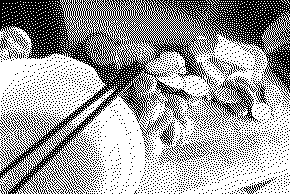图 2 mode = '1'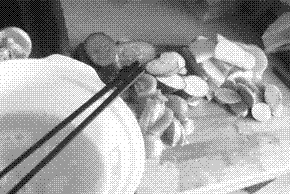图 3 mode = 'L'图 4 mode = 'P'
convert() 函数也接受另一个隐含参数 matrix，转换矩阵 matrix 是一个长度为4 或者16 tuple。下例是一个转换 RGB 空间到 CIE XYZ 空间的例子：
rgb2xyz = (
0.412453, 0.357580, 0.180423, 0,
0.212671, 0.715160, 0.072169, 0,
0.019334, 0.119193, 0.950227, 0 )
out = im.convert("RGB", rgb2xyz)
除了完备的色彩空间转换能力外， PIL 还提供了resize()、rotate()等函数以获得改变大小，旋转图片等几何变换能力，在图像识别方面，图像实例提供了一个 histogram() 方法来计算直方图，非常方便实用。
图像增强
图像增强通常用以图像识别之前的预处理，适当的图像增强能够使得识别过程达到事半功倍的效果。 PIL 在这方面提供了一个名为 ImageEnhance 的模块，提供了几种常见的图像增强方案：
import ImageEnhance
enhancer = ImageEnhance.Sharpness(image)
for i in range(8):
factor = i / 4.0
enhancer.enhance(factor).show("Sharpness %f" % factor)
上面的代码即是一个典型的使用 ImageEnhance 模块的例子。 Sharpness 是 ImageEnhance 模块的一个类，用以锐化图片。这一模块主要包含如下几个类：Color、Brightness、Contrast和Sharpness。它们都有一个共同的接口 .enhance(factor) ，接受一个浮点参数 factor，标示增强的比例。下面看看这四个类在不同的 factor 下的效果图 5 使用Color 进行色彩增强，factor 取值 [0, 4]，步进 0.5图 6 用 Birghtness 增强亮度，factor取值[0,4]，步进0.5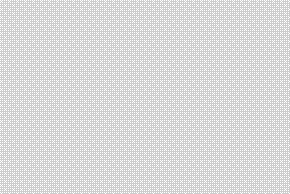图 7用 Contrast 增强对比度， factor 取值 [0,4]，步进0.5图 8用 Sharpness 锐化图像，factor取值 [0,4]，步进0.5
图像 Filter
PIL 在 Filter 方面的支持是非常完备的，除常见的模糊、浮雕、轮廓、边缘增强和平滑，还有中值滤波、ModeFilter等，简直方便到可以做自己做一个Photoshop。这些 Filter 都放置在 ImageFilter 模块中，ImageFilter主要包括两部分内容，一是内置的 Filter，如 BLUR、DETAIL等，另一部分是 Filter 函数，可以指定不同的参数获得不同的效果。示例如下：
import ImageFilter
im1 = im.filter(ImageFilter.BLUR)
im2 = im.filter(ImageFilter.MinFilter(3))
im3 = im.filter(ImageFilter.MinFilter()) # same as MinFilter(3)
可以看到 ImageFilter 模块的使用非常简单，每一个 Filter 都只需要一行代码就可调用，开发效率非常高。图 9使用 BLUR图 10使用 CONTOUR图 11使用 DETAIL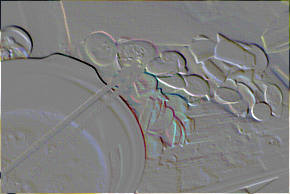图 12使用 EMBOSS图 13使用 EDGE_ENHANCE图 14使用 EDGE_ENHANCE_MORE图 15使用 FIND_EDGES图 16使用 SHARPEN图 17使用 SMOOTH图 18使用 SMOOTH_MORE
以上是几种内置的 Filter 的效果图，除此之外， ImageFilter 还提供了一些 Filter 函数，下面我们来看看这些可以通过参数改变行为的 Filter 的效果：图 19使用 Kernel()，参数：size = (3, 3), kernel = (0.5, 0.5, 0.5, 0.5, 0.5, 0.5, 0.5, 0.5, 0.5)图 20使用 MaxFilter，默认参数图 21使用 MinFilter，默认参数图 22使用 MedianFilter，默认参数图 23使用 ModeFilter，参数 size = 3图 24使用 RankFilter，参数 size = 3, rank = 3
小结
到此，对 PIL 的介绍就告一段落了。总的来说，对于图像处理和识别，PIL 内建了强大的支持，从各种增强算法到 Filter ，都让人无法怀疑使用 Python 的可行性。 Python唯一的劣势在于执行时间过慢，特别是当实现一些计算量大的算法时候，需要极强的耐心。我曾用 Hough Transform（霍夫变换）来查找图像中的直线，纯 Python 的实现处理一个 340 * 100 的图片也要花去数秒时间（P4 3.0G + 1G memory）。但使用 PIL 无需关注图像格式、内建的图像增强算法和 Filter 算法，这些优点使 Python 适合用于构造原型和进行实验，在这两方面Python 比 matlab 更加方便。商业的图像识别产品开发，可以考虑已经被 boost accepted的来自 adobe 的开源 C++ 库 gil，可以兼顾执行性能和开发效率。
id="alimamaifrm" style="WIDTH: 750px; HEIGHT: 110px" border="0" name="alimamaifrm" marginwidth="0" marginheight="0" src="http://p.alimama.com/cpacode.php?t=A&pid=mm_10108440_0_0&w=750&h=110&rn=1&cn=3&ky=%CA%E9&cid=50000072&bgc=FFFFFF&bdc=E6E6E6&tc=0000FF&dc=000000" frameborder="0" width="750" scrolling="no" height="110">
展开全文python 图像处理 filter import image
• 本文讲述在Python中对...首先，import cv2，import os，from PIL import Image，接下来让我们愉快地进行图像处理操作。 #读取图像 img = cv2.imread(os.path.join(im_dir, im_path + '.jpg')) #图像融合 img2 = img*0.python 图像处理
• 图像处理是分析和操纵数字图像的过程，旨在提高其质量或从中提取一些信息，然后将其用于某些方面。 图像处理中的常见任务包括显示图像，基本操作（如裁剪、翻转、旋转等），图像分割，分类和特征提取，图像恢复和...
• 提到图像处理第一个想到的库就是PIL，全称Python Imaging Library Python图像处理类库，它提供了大量的图像操作，比如图像缩放，裁剪，贴图，模糊等等，很多时候它需要配合numpy库一起使用 1.open() 你可以使用...python 图像处理
• Python 图像简单处理的几个常用的库 #Python 图像简单处理的几个常用的库 你好！ 这是博主今日简单学习的Python图像简单处理的几个常用库，第一次发的博客，有些简陋，以后会精进 Image（PIL） Image.open()返回一...Python 图像处理
• ) ，OpenCV目前支持读取bmp、jpg、png、tiff等常用格式。 创建一个窗口 cv2.namedWindow("Image")  然后在窗口中显示图像 cv2.imshow("Image", img)  cv2.waitKey (0)  cv2....
• 该系列文章是讲解Python OpenCV图像处理知识，前期主要讲解图像入门、OpenCV基础用法，中期讲解图像处理的各种算法，包括图像锐化算子、图像增强技术、图像分割等，后期结合深度学习研究图像识别、图像分类应用。...Python 图像处理 OpenCV
• Python图像处理  最近在做一件比较 evil 的事情——验证码识别，以此来学习一些新的技能。...在 Python 中，比较常用图像处理库是 PIL(Python Image Library)，当前版本是 1.1.6 ，用起来非常方便。大家可
• 这些Python库提供了一种简单直观的方法来转换图像并理解底层数据。 今天的世界充满了数据，图像是这些数据的重要组成部分。但是，在使用它们之前，必须...Python是这些图像处理任务的绝佳选择，因为它作为一种科学...
• PillowPython平台的图像处理标准库获取图片大小运行示例#!/usr/bin/env python3 # -*- coding: utf-8 -*- # Python基础 Pillowfrom PIL import Imagedef getSize(): # 打开一个图片 pic = Image.open("D:/Python...python 图像处理 PIL
• python数字图像处理-图像噪声与去噪算法图像噪声椒盐噪声概述： 椒盐噪声（salt & pepper noise）是数字图像的一个常见噪声，所谓椒盐，椒就是黑，盐就是白，椒盐噪声就是在图像上随机出现黑色白色的像素。...python 图像处理 算法
• 本篇文章讲解图像灰度化处理的知识，结合OpenCV调用cv2.cvtColor()函数实现图像灰度操作，使用像素处理方法对图像进行灰度化处理。基础性知识希望对您有所帮助。 1.图像灰度化原理 2.基于OpenCV的图像灰度化处理 3....Python 图像处理 OpenCV 灰度化处理
...